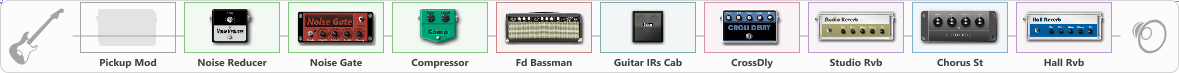# Good Clean

Discussion in 'ToneLib-GFX presets' started by sopu, Jul 21, 2022.

1. Good Clean

Preset name: Chorused clean tone

Effects chain:Effect: "Pickup Mod" (Dynamics / Filter), active - "no"
{
"Mode" = Humbacker->Single
"Tone" = 56
}

Effect: "Noise Reducer" (Dynamics / Filter), active - "yes"
{
"Sens" = 100
"Mode" = Hard
}

Effect: "Noise Gate" (Dynamics / Filter), active - "yes"
{
"Mode" = Manual
"Depth" = 100
"Threshold" = 100
"Attack" = 500
"Hold" = 1593
"Decay" = 2000
}

Effect: "Compressor" (Dynamics / Filter), active - "yes"
{
"Sense" = 31
"Level" = 35
}

Effect: "Fd Bassman" (Amp simulators), active - "yes"
{
"Gain" = 4
"Bass" = 63
"Middle" = 65
"Treble" = 68
"Presence" = 54
"Master" = 58
"Level (dB)" = 20
}

Effect: "Guitar IRs Cab" (Cabinets), active - "yes"
{
"Model" = Fender Twin Rev (2x12")
"Mic Position" = Edge
"Mic Distance" = Near
"Low Cut (Hz)" = 60
"Hi Cut (kHz)" = 19.5
"Mix" = 100
"Level (dB)" = 17
}

Effect: "CrossDly" (Delay), active - "yes"
{
"Time" = 36
"Feedback" = 0
"Tone" = 58
"Sens" = 0
"Mix" = 24
}

Effect: "Studio Rvb" (Reverberation), active - "yes"
{
"Time" = 7.4
"PreDelay" = 32
"LoDamp" = 0
"HiDamp" = 12
"Mix" = 18
}

Effect: "Chorus St" (Modulation / Sfx), active - "yes"
{
"Speed" = 3.2
"Depth" = 51
"Center" = 6.4
"Mix" = 100
}

Effect: "Hall Rvb" (Reverberation), active - "yes"
{
"Time" = 9.4
"PreDelay" = 61
"LoDamp" = 49
"HiDamp" = 17
"Mix" = 28
}

Note: You will need to download and install the ToneLib-GFX software to use the preset.

File size:
963 bytes
Views:
2,080